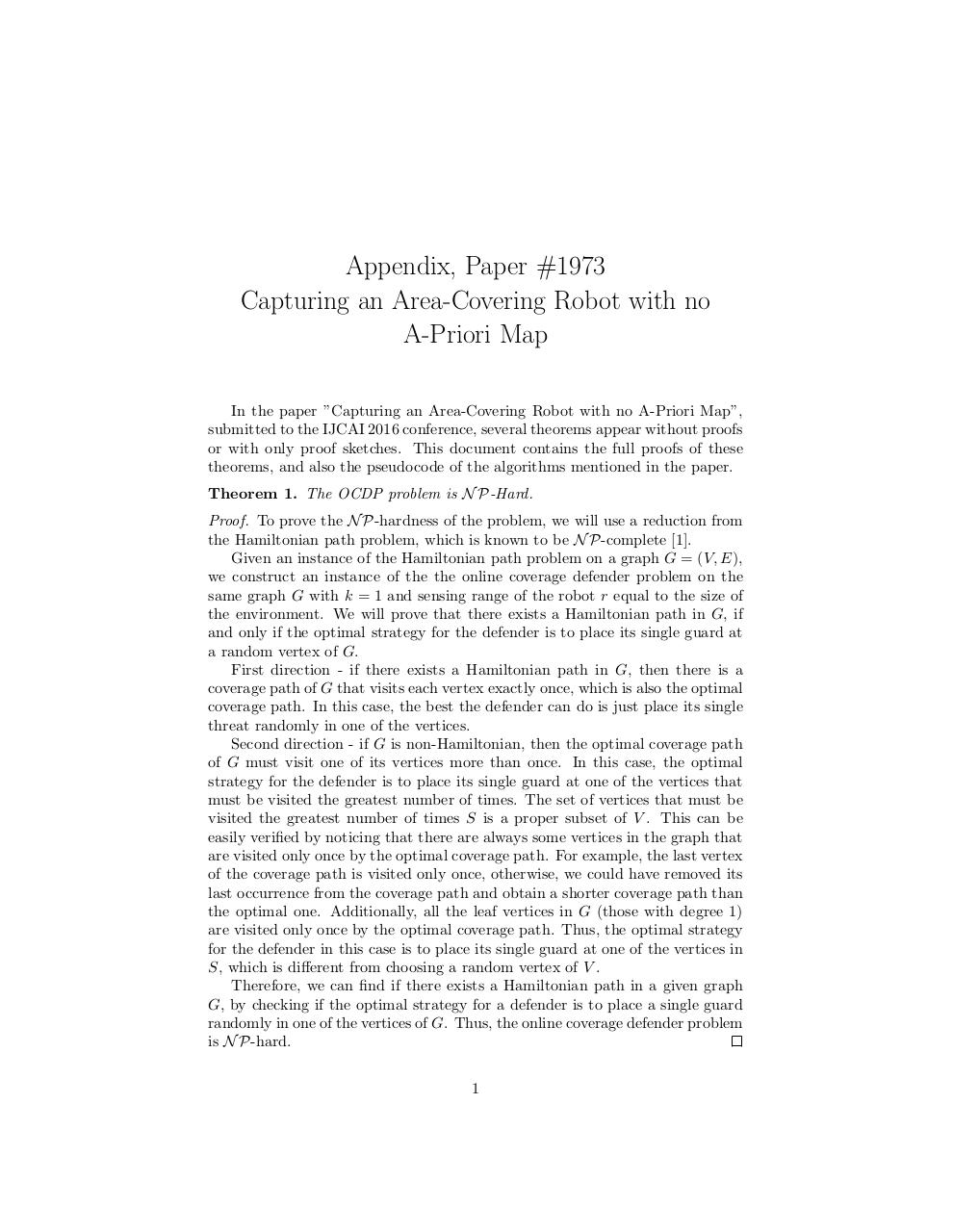Page 1 2 3 4 5 6 7 8 9 10

#### Text preview

Appendix, Paper #1973
Capturing an Area-Covering Robot with no
A-Priori Map
In the paper ”Capturing an Area-Covering Robot with no A-Priori Map”,
submitted to the IJCAI 2016 conference, several theorems appear without proofs
or with only proof sketches. This document contains the full proofs of these
theorems, and also the pseudocode of the algorithms mentioned in the paper.
Theorem 1. The OCDP problem is N P-Hard.
Proof. To prove the N P-hardness of the problem, we will use a reduction from
the Hamiltonian path problem, which is known to be N P-complete .
Given an instance of the Hamiltonian path problem on a graph G = (V, E),
we construct an instance of the the online coverage defender problem on the
same graph G with k = 1 and sensing range of the robot r equal to the size of
the environment. We will prove that there exists a Hamiltonian path in G, if
and only if the optimal strategy for the defender is to place its single guard at
a random vertex of G.
First direction - if there exists a Hamiltonian path in G, then there is a
coverage path of G that visits each vertex exactly once, which is also the optimal
coverage path. In this case, the best the defender can do is just place its single
threat randomly in one of the vertices.
Second direction - if G is non-Hamiltonian, then the optimal coverage path
of G must visit one of its vertices more than once. In this case, the optimal
strategy for the defender is to place its single guard at one of the vertices that
must be visited the greatest number of times. The set of vertices that must be
visited the greatest number of times S is a proper subset of V . This can be
easily verified by noticing that there are always some vertices in the graph that
are visited only once by the optimal coverage path. For example, the last vertex
of the coverage path is visited only once, otherwise, we could have removed its
last occurrence from the coverage path and obtain a shorter coverage path than
the optimal one. Additionally, all the leaf vertices in G (those with degree 1)
are visited only once by the optimal coverage path. Thus, the optimal strategy
for the defender in this case is to place its single guard at one of the vertices in
S, which is different from choosing a random vertex of V .
Therefore, we can find if there exists a Hamiltonian path in a given graph
G, by checking if the optimal strategy for a defender is to place a single guard
randomly in one of the vertices of G. Thus, the online coverage defender problem
is N P-hard.
1